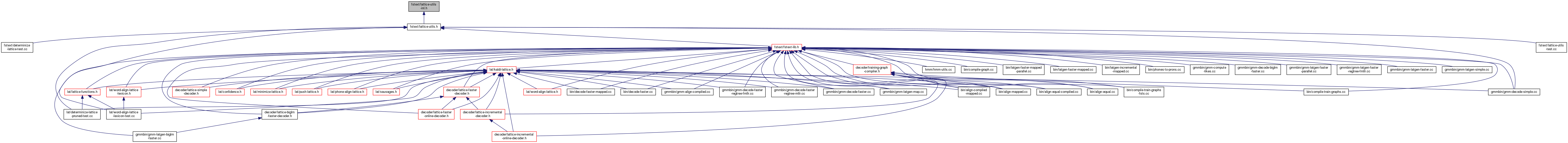lattice-utils-inl.h File Reference
This graph shows which files directly or indirectly include this file:Go to the source code of this file.

## Namespaces

fst
For an extended explanation of the framework of which grammar-fsts are a part, please see Support for grammars and graphs with on-the-fly parts. (i.e.

## Functions

template<class Weight , class Int >
void ConvertLattice (const ExpandedFst< ArcTpl< Weight > > &ifst, MutableFst< ArcTpl< CompactLatticeWeightTpl< Weight, Int > > > *ofst, bool invert=true)
Convert lattice from a normal FST to a CompactLattice FST. More...

template<class Weight , class Int >
void ConvertLattice (const ExpandedFst< ArcTpl< CompactLatticeWeightTpl< Weight, Int > > > &ifst, MutableFst< ArcTpl< Weight > > *ofst, bool invert=true)
Convert lattice CompactLattice format to Lattice. More...

template<class WeightIn , class WeightOut >
void ConvertLattice (const ExpandedFst< ArcTpl< WeightIn > > &ifst, MutableFst< ArcTpl< WeightOut > > *ofst)
Convert between CompactLattices and Lattices of different floating point types... More...

template<class Weight , class ScaleFloat >
void ScaleLattice (const std::vector< std::vector< ScaleFloat > > &scale, MutableFst< ArcTpl< Weight > > *fst)
Scales the pairs of weights in LatticeWeight or CompactLatticeWeight by viewing the pair (a, b) as a 2-vector and pre-multiplying by the 2x2 matrix in "scale". More...

template<class Weight , class Int >
void RemoveAlignmentsFromCompactLattice (MutableFst< ArcTpl< CompactLatticeWeightTpl< Weight, Int > > > *fst)
Removes state-level alignments (the strings that are part of the weights). More...

template<class Weight , class Int >
bool CompactLatticeHasAlignment (const ExpandedFst< ArcTpl< CompactLatticeWeightTpl< Weight, Int > > > &fst)
Returns true if lattice has alignments, i.e. More...

template<class Real >
void ConvertFstToLattice (const ExpandedFst< ArcTpl< TropicalWeight > > &ifst, MutableFst< ArcTpl< LatticeWeightTpl< Real > > > *ofst)
Converts TropicalWeight to LatticeWeight (puts all the weight on the first float in the lattice's pair). More...